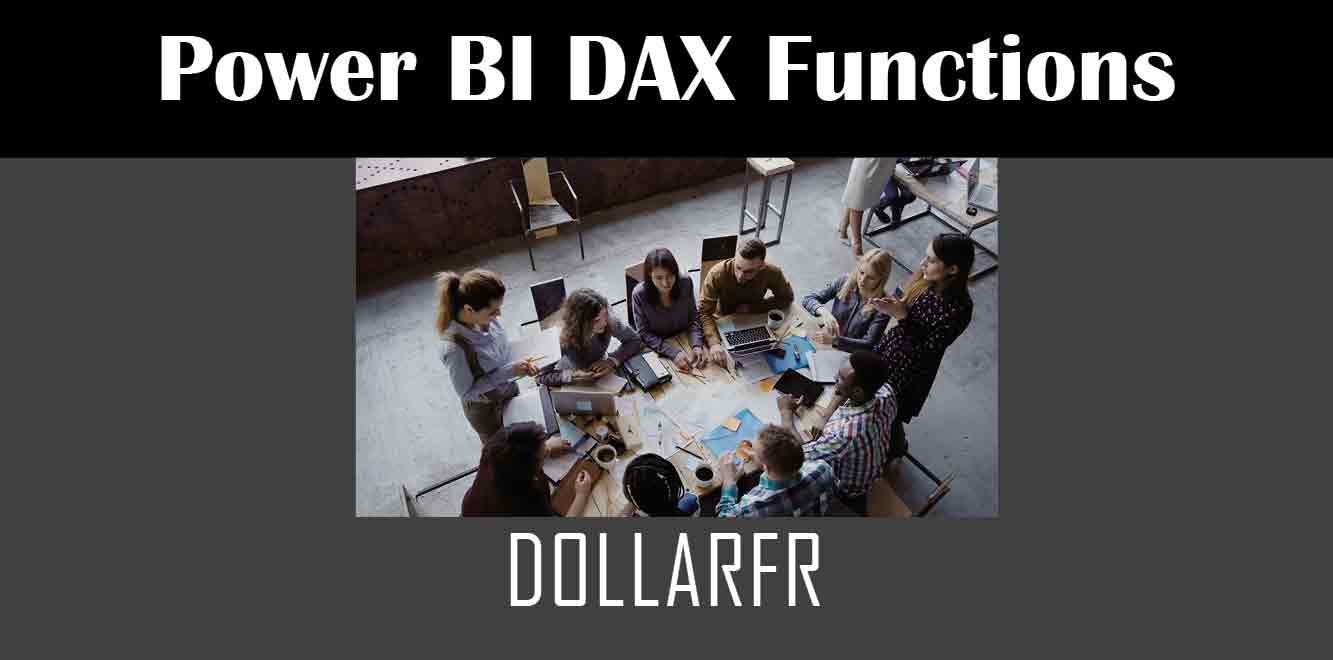# DOLLARFR

## Y## Syntax of the DOLLARFR Function

The syntax of the DOLLARFR function is as follows:

DOLLARFR(fractional_dollar, denominator)

``` Where: * `fractional_dollar`: This is the fractional dollar value that you want to convert to a dollar value. * `denominator`: This is the denominator value that is used to calculate the fraction of the dollar. For example, if the fractional dollar value is 45/64, the denominator value would be 64. Example of Using the DOLLARFR Function Let's look at an example of how to use the DOLLARFR function in Power BI. Suppose we have a table of sales data that contains fractional dollar values, and we want to convert these values into dollar values. The table looks like this: | Sale ID | Sale Amount | |--------|------------| | 1 | 35/64 | | 2 | 19/32 | | 3 | 51/128 | | 4 | 21/64 | To convert the fractional dollar values into dollar values, we can create a new measure using the DOLLARFR function. To do this, follow these steps: 1. Open the Power BI desktop application and navigate to the Data View. 2. Right-click on the table that contains the fractional dollar values and select "New Measure". 3. In the formula bar, enter the following formula: ```

Dollar Value = DOLLARFR(‘Sales'[Sale Amount], 64)

``` This formula tells Power BI to use the DOLLARFR function to convert the fractional dollar values in the "Sale Amount" column of the "Sales" table into dollar values, using a denominator of 64. 4. Click "Enter" to create the new measure. Once the new measure has been created, we can add it to a visual to display the dollar values. To do this, follow these steps: 1. Navigate to the report view in Power BI. 2. Drag and drop the "Sale ID" and "Dollar Value" measures onto a table visual. 3. Power BI will automatically format the "Dollar Value" column as a currency, displaying the dollar symbol and two decimal places. In conclusion, the DOLLARFR function is a useful tool for converting fractional dollar values into dollar values in financial analysis. By using this function in Power BI, you can create custom calculations and visualizations that display dollar values in a clear and concise way. Power BI DAX Training Courses by G Com Solutions (0800 998 9248)Power BI DAX Intensive Training Course £1,050.00 – £26,550.00 Select optionsContinue Loading DonePower BI DAX Introduction £395.00 – £9,750.00 Select optionsContinue Loading DonePower BI DAX Intermediate £395.00 – £9,750.00 Select optionsContinue Loading DonePower BI DAX Advanced £395.00 – £9,750.00 Select optionsContinue Loading Done Upcoming Courses Contact Us Subject Your Name (required) Company/Organisation Email (required) Telephone Training Course(s) Power BI Intensive TrainingPower BI introduction Power BI IntermediatePower BI AdvancedDAXPower Query MPower BI CertificationPower BI AdministrationPower PlatformPower AutomatePower AppsOTHER Your Message Upload Example Document(s) (Zip multiple files) ```
``` ```
``` ```
``` ```
``` ```
``` ```